Next: An example 1D PIC Up: Particle-in-cell codes Previous: Evaluation of electron number

## Solution of Poisson's equation

Consider the solution of Poisson's equation: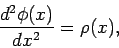(303)

where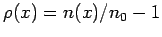. Note that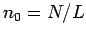in normalized units. Let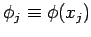and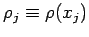. We can write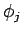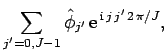(304)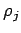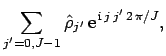(305)

which automatically satisfies the periodic boundary conditions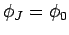and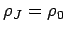. Note that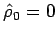, since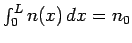. The other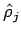are obtained from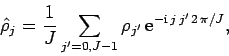(306)

for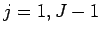. The Fourier transformed version of Poisson's equation yields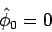(307)

and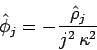(308)

for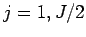, where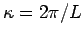. Finally,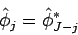(309)

for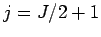to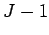, which ensures that theremain real. The discretized version of Eq. (297) is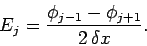(310)

Of course,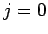and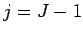are special cases which can be resolved using the periodic boundary conditions. Finally, suppose that the coordinate of the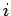th electron lies between the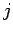th andth grid-points: i.e.,. We can then use linear interpolation to evaluate the electric field seen by theth electron: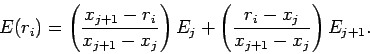(311)Next: An example 1D PIC Up: Particle-in-cell codes Previous: Evaluation of electron number
Richard Fitzpatrick 2006-03-29